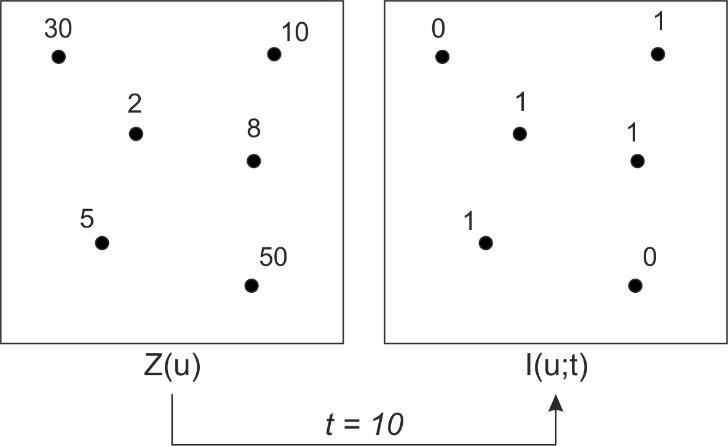# Indicator Random Variable

## What is an Indicator Random Variable?

In mathematics, variables are used to define unknown values in a function or expression. An Indicator Random Variable is a particular kind of variable that specifically represents whether or not an occurrence happened. Accordingly, the values for the Indicator Random Variable are limited to 1 and 0, in which 1 represents the occurrence of the event, and 0 represents the lack of occurrence.

## How does an Indicator Random Variable work?

Because the Indicator Random Variable is associated with the occurrence of an event, it is a particularly handy component when working with layered derivations. To better understand how the Indicator Random Variable works, it is helpful to use an example. Imagine we are trying to find the sum of a set of  "N" throws of a die. Since the amount of throws is set as unknown at this point, the sum of all the throws cannot yet be calculated without an Indicator Random Variable. If we assign the variable a value of one, indicating the event happening, we can compute the expected value of a single die by adding up all the values of the die and dividing it by the number of sides (i.e. 3.5). Naturally, the sum of "N" throws of the die will be "N" multiplied by 3.5, or simply 3.5N.Source

The image above is a graphical representation of the process of translating a set of input data into indicator random variables, given a set threshold, in this case 10. Accordingly, if the value of t is less than or equal to 10, the indicator random variable is 1, otherwise it is 0.